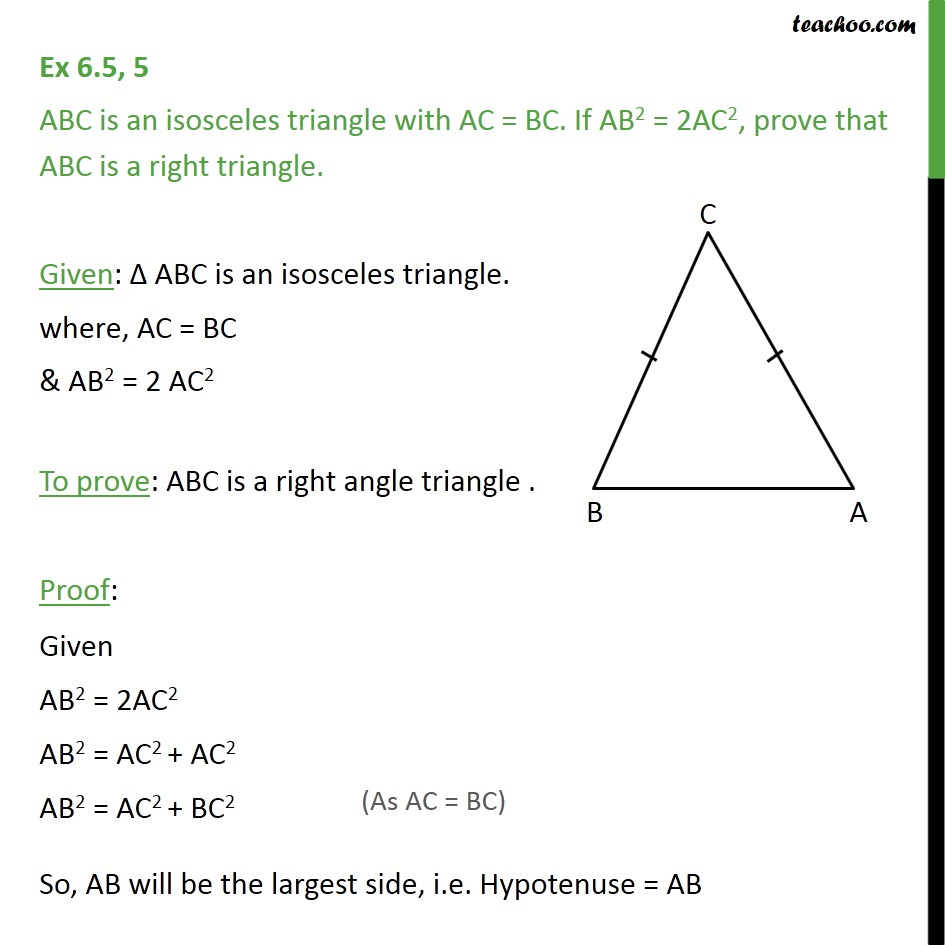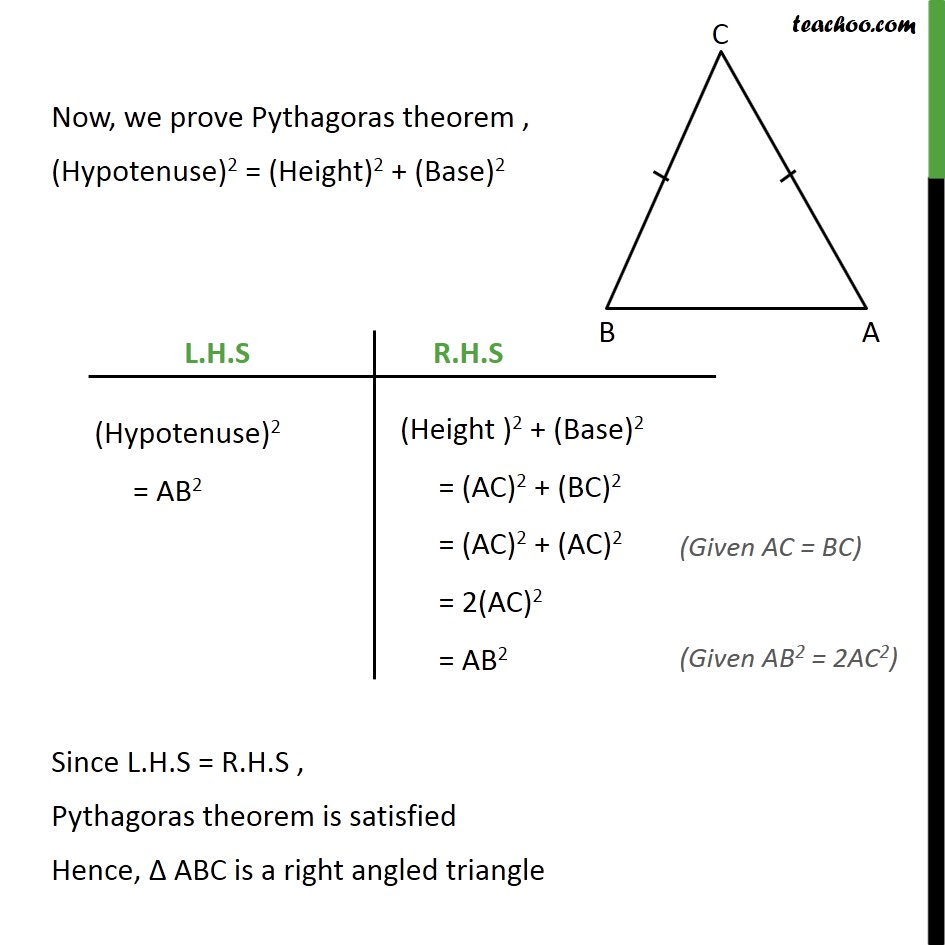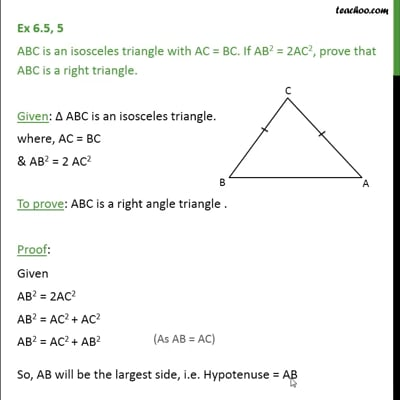Ex 6.5

Chapter 6 Class 10 Triangles
Serial order wiseThis video is only available for Teachoo black users

Solve all your doubts with Teachoo Black (new monthly pack available now!)

### Transcript

Ex 6.5, 5 ABC is an isosceles triangle with AC = BC. If AB2 = 2AC2, prove that ABC is a right triangle. Given: ABC is an isosceles triangle. where, AC = BC & AB2 = 2 AC2 To prove: ABC is a right angle triangle . Proof: Given AB2 = 2AC2 AB2 = AC2 + AC2 AB2 = AC2 + BC2 So, AB will be the largest side, i.e. Hypotenuse = AB Now, we prove Pythagoras theorem , (Hypotenuse)2 = (Height )2 + (Base)2 L.H.S (Hypotenuse)2 = AB2 R.H.S (Height )2 + (Base)2 = (AC)2 + (BC)2 = (AC)2 + (AC)2 (Given AC = BC) = 2(AC)2 = AB2 (Given AB2 = 2AC2) Since L.H.S = R.H.S , Pythagoras theorem is satisfied Hence ABC is a right angled triangle